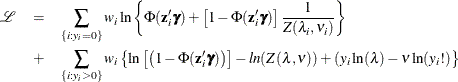# The COUNTREG Procedure

### Zero-Inflated Conway-Maxwell-Poisson Regression

In the Conway-Maxwell-Poisson regression model, the data generation process is defined as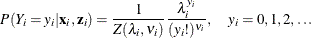where the normalization factor is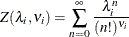and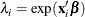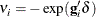The zero-inflated Conway-Maxwell-Poisson model can be written as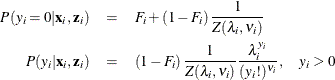The conditional expectation and conditional variance ofare given by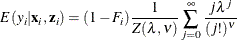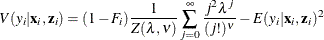General form of the log-likelihood function for the Conway-Maxwell-Poisson zero-inflated model is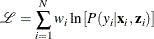#### Zero-Inflated Conway-Maxwell-Poisson Model with Logistic Link Function

In this model the probability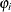is expressed with a logistic link function as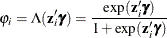The log-likelihood function is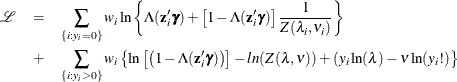#### Zero-Inflated Conway-Maxwell-Poisson Model with Normal Link Function

For this model, the probabilityis specified with the standard normal distribution function (probit function):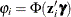.

The log-likelihood function is written as